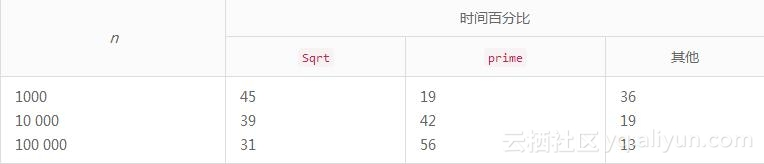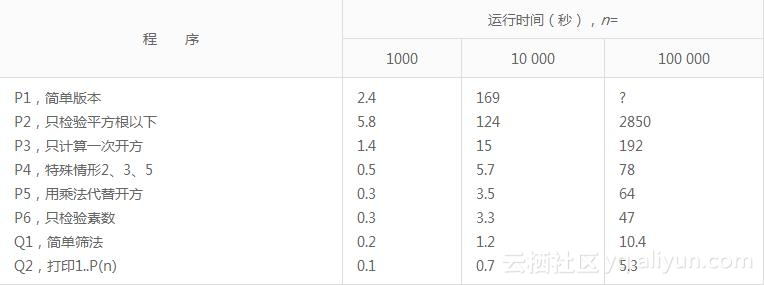#《编程珠玑（续）（修订版）》—第1章1.1节计算素数

0
0
01. 云栖社区>
2. 博客>
3. 正文

## 《编程珠玑（续）（修订版）》—第1章1.1节计算素数

1.1　计算素数

javascript
int prime(int n)
{　　int i;
999　　　　 for (i = 2; i < n; i++)
78022　　　　　　if(n%i == 0)
831　　　　　　　　 return 0;
168　　　　 return 1;
}
main()
{　　int i, n；
1　　　　　　n = 1000；
1　　　　　　for (i = 2; i <= n; i++)
999　　　　　　　if (prime(i))
168　　　　　　　　 printf("%dn", i);
}

如果整型参数n是素数，上述prime函数返回1（真），否则返回0。这个函数检验2到n-1之间的所有整数，看其是否整除n。上述main过程用prime子程序来依次检查整数2～1000，发现素数就打印出来。

831=999）。prime函数共测试了78022个可能的因子，或者说为了确定素数性，对每个整数检查了大约78个因子。



int root(int n)
5456　　 { return (int) sqrt((float) n); }

int prime(int n)
{　 int i;
999　　　　　for (i = 2;　i < = root(n);　i++)
5288　　　　　　 if (n % i == 0)
831　　　　　　　　　 return 0;
168　　　　　return 1;
}

main()
{　　int i, n;
1　　　　　　 n = 1000;
1　　　　　　 for (i = 2;　i < = n;　i++)
999　　　　　　　 if (prime(i))
168　　　　　　　　　　printf("%dn", i);
}

修改显然是有效的：程序P2的行计数显示，只测试了5288个因子（程序P1的1/14），总共调用了5456次root（测试了5288次整除性，168次由于i超出了root(n)而终止循环）。不过，虽然计数大大减少了，但是程序P2运行了5.8秒，而程序P1只运行了2.4秒（本节末尾的表中含有运行时间的更多细节）。这说明什么呢？



%time　　cumsecs　　 　　#call　　　　ms/call　　　　　 name
82.7　　　　4.77　　　　　　　　　　　　　　　　　　　　_sqrt
4.5　　　　5.03　　　　　999　　　　　 0.26　　　 　　 _prime
4.3　　　　5.28　　　　 5456　　　　　 0.05　　　 　　 _root
2.6　　　　5.43　　　　　　　　　　　　　　　　　　　　_frexp
1.4　　　　5.51　　　　　　　　　　　　　　　　　　　　_ _doprnt
1.2　　　　5.57　　　　　　　　　　　　　　　　　　　 _write
0.9　　　　5.63　　　　　　　　　　　　　　　　　　　　mcount
0.6　　　　5.66　　　　　　　　　　　　　　　　　　　　_creat
0.6　　　　5.69　　　　　　　　　　　　　　　　　　　　_printf
0.4　　　　5.72　　　　　　 1　　　　 25.00　　　 　　 _main
0.3　　　　5.73　　　　　　　　　　　　　　　　　　　　_close
0.3　　　　5.75　　　　　　　　　　　　　　　　　　　　_exit
0.3　　　　5.77　　　　　　　　　　　　　　　　　　　　_isatty

过程按照运行时间递减的顺序列出。时间上既显示出总秒数，也显示出占总时间的百分比。编译后记录下源程序中main、prime和root这3个过程的调用次数。再次看到这几个计数是令人鼓舞的。其他过程没有性能监视的库函数，完成各种输入/输出和清理维护工作。第4列说明了带语句计数的所有函数每次调用的平均毫秒数。

javascript
int prime(int n)
{　　int i, bound;
999　　　　　 bound = root(n);
999　　　　　 for (i = 2;　i < = bound;　i++)
5288　　　　　　　 if (n % i == 0)
831　　　　　　　　　　 return 0;
168　　　　　　　　return 1;
}


100 000的情形为例，过程时间性能监视显示，sqrt占用了程序P2的88%的运行时间，但是只占用了程序P3的48%的运行时间。这好多了，但仍然是循环的累赘。

int root(int n)
265　　  {　　return (int) sqrt((float) n);　}

int prime(int n)
{　　int i, bound;
999　　　　　 if (n % 2 == 0)
500　　　　　　　 return 0;
499　　　　　 if (n % 3 == 0)
167　　　　　　　 return 0;
332　　　　　 if (n % 5 == 0)
67　　　　　　　　return 0;
265　　　　　 bound = root(n);
265　　　　　 for (i = 7; i <= bound; i = i+2)
1530　　　　　　　 if (n % i == 0)
100　　　　　　　　　　 return 0;
165　　　　　 return 1;
}
main()
{　　　int i, n;
1　　　　　　 n = 1000;
1　　　　　　 for (i = 2;　i <= n; i++)
999　　　　　　　　if (prime(i))
165　　　　　　　　　　printf("%d\n", i);
}


if (n % 2 == 0)
return (n　== 2);265　　　　　　 for　(i　= 7;　i*i　<= n;　i　= i+2)
1530　　　　　　　　　if (n % i == 0)
100　　　　　　　　　　　　return 0;
165　　　　　　 return 1;+ 关注